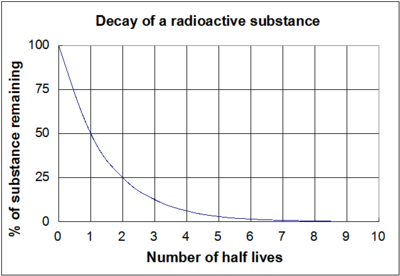# Half lifeFigure 1. A chart showing the decay of a radioactive nucleus over time. The time that it takes the mass or activity of the source (the number of decay events per second) to fall to the 50% mark is the half life. The half life in this image is 1 year.

Half life is the time that it takes for half of the original value of some amount of a radioactive element to decay. Additionally, one half life is the time that it takes for the activity of a source to fall to half its original value. The second law of thermodynamics, specifically the disorder statement can be used to help understand why radioactive decay occurs. This statement says that the entropy of a closed system can never decrease, meaning that things must fall further into disorder, not order. This process is known as "decay" and the second law helps to clarify why matter breaks down into a less and less organized state over time this . Part of this process includes certain types of atoms which break down into new, different types of atoms at some measurable rate known as radioactive decay.

All radioactive materials have unstable nuclei within them. Additionally, there are also some nuclei within the substance that are already in their stable state but the proportion of stable to unstable nuclei in a sample can vary. The stable nuclei in the sample are unchanging (and in a stable energetic state), but the unstable nuclei will undergo some sort of nuclear decay over time to become stable. This results in an emission of some form of radiation. Since half life is a measure of time, the half life is a value that determines how long this reduction to a more stable energy state will take.

Different substances experience a loss of their radioactivity more quickly than others. Some radioactive elements can have half of their unstable nuclei decay in less than one second. For example, krypton-101 has a half life of about a ten millionth of a second. In contrast, some elements have extraordinarily long half lives and take billions of years to decay. Uranium-238 has a half life of 4.51 billion years. This means that it would take billions of years for uranium-238 to decay into a ratio of half uranium-238 and half thorium-234. Uranium-235 (another naturally occurring isotope of uranium) has a shorter half life than uranium-238, that's only ~700 million years.

## Equation

There is an equation that is frequently used to determine how much of a certain radioactive substance remains after a given time has passed. This is determined from properties such as the half life of the substance, and how much of the substance there was initially. The equation used is:

where:

• is the amount of substance after time has passed
• is the initial amount of substance
• is the amount of time that has passed
• is the half life of the substance

Additionally, a similar equation can be used to show how the activity of the substance diminishes over time. When this is being expressed, the equation takes the form:

where:

• is the activity of the substance after time has passed
• is the initial activity of the substance
• is the amount of time that has passed
• is the half life of the substance

The graph shown in Figure 1 is a visual representation of these equations above. It is important to note that regardless of the actual length of the half-life (whether it is millions of years or a few nanoseconds) the shape of the graph will be the same.

Knowledge of half lives is part of how geologists date rocks with radioisotopic dating.

## Authors and Editors

Bethel Afework, Monica Friesen, Jordan Hanania, Braden Heffernan, James Jenden, Kailyn Stenhouse, Jason Donev
Last updated: May 18, 2018
Get Citation